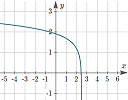# Posts tagged ‘Graphs’

## IntMath Newsletter: Farewell, online math resources

0. Fond farewell
1. Math in the news
2. Resource: ClassCalc
3. Math movie
4. Math art and code
5. Math puzzle
6. Final thought: Reset

## IntMath Newsletter: Desmos competition, resources, math movies

1. Competition: Desmos
2. Resources: Wolfram, Worldometers
3. Math in the news: Trendlines, photos
4. Math movies: Irrational, Hollywood math
5. Math puzzles
6. Final thought: Hot air

2. Resources: Desmos, e-book
3. Math in the news: Review and teaspoon
4. Math movies: Coin, key
5. Math puzzle: Race
6. Final thought: Real cost

## IntMath Newsletter: Putnam, review, Captive, cipher

0. Season's greetings
1. New on IntMath: Putnam
2. The year in review
3. Math movies: Captive
4. Math puzzle: Cipher
5. Final thought: Imagine

## Intmath Newsletter: Poly equations, charts, potential, water

1. New on IntMath: Equations graph solver
2. Resources: Charts
3. Math in the news: Lenses, integral equations
4. Math movies: Potential
5. Math puzzle: Age
6. Final thought: Water

## IntMath Newsletter: Calculators, atomic clocks, tensors

1. On IntMath: Calculators
2. Music lab, Webinars
3. AI, atomic clocks
4. Tensor, irrationals
5. Math puzzle
6. They knew

## IntMath Newsletter: CSS matrix, Humble Pi, van Gogh

1. New on IntMath: CSS matrix math
3. Math in the news: Proof
4. Math movies: Parker, van Gogh
5. Math puzzle: Mystery object
6. Final thought: Dry leaves

## IntMath Newsletter: Eigenvectors, 3D solar system, graphics

1. New on IntMath: Eigenvalues and eigenvectors
2. Resources: Desmos and 3D simulator
3. Math in the news: News and science
4. Math movies: Graphics and addresses
5. Math puzzle: Tangent circles
6. Final thought: Culture

## IntMath Newsletter: Log graphs, challenges, news

1. New on IntMath: Logarithm function from its graph
2. Breakthrough Junior Challenge
2. Resources: Wolfram, MathGraph32
3. Math in the news: Computers and equations
4. Math movies: Calculus, Probability
5. Math puzzle: Bowling
6. Final thought: extinctions

## How to find the equation of a logarithm function from its graph?This article explains how to determine the equation of a logarithmic function given its graph

## Full archive

See the blog sitemap for all previous articles.